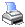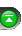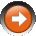﻿ R-squared
 NinjaScript > Language Reference > Indicator Methods > R-squaredDescription

The r-squared indicator calculates how well the price approximates a linear regression line. The indicator gets its name from the calculation, which is, the square of the correlation coefficient (referred to in mathematics by the Greek letter rho, or r). The range of the r-squared is from zero to one.

... Courtesy of FMLabs

Syntax

 RSquared(int period) RSquared(IDataSeries input, int period)   Returns default value RSquared(int period)[int barsAgo] RSquared(IDataSeries input, int period)[int barsAgo]

Return Value

double; Accessing this method via an index value [int barsAgo] returns the indicator value of the referenced bar.

Parameters

 input Indicator source data (?) period Number of bars used in the calculation

Examples

 // Prints the current value of a 20 period R-squared using default price type double value = RSquared(20); Print("The current R-squared value is " + value.ToString());   // Prints the current value of a 20 period R-squared using high price type double value = RSquared(High, 20); Print("The current R-squared value is " + value.ToString());

Source Code

You can view this indicator method source code by selecting the menu Tools > Edit NinjaScript > Indicator within the NinjaTrader Control Center window.